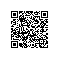# 斯坦福-随机图模型-week3.3_

title: 斯坦福-随机图模型-week3.3
tags: note
notebook: 6- 英文课程-9-Probabilistic Graphical Models 1: Representation
---

# 斯坦福-随机图模型-week3.3

## 1. Question 1

I-Maps. Graph G (shown below) is a perfect I-map for distribution P, i.e. I(G)=I(P). Which of the other graphs is an I-map (not necessarily a perfect map) for P?

III

Correct
I isn't because it has the extra independence (A⊥C).

II has the extra independence relation (B⊥C∣D) (among others).

III has no extra independencies. In fact, it has fewer independencies, but the definition of I-map allows for this.

II and III

I

I and II

### Question 2

I-Equivalence. In the figure below, graph G is I-equivalent to which other graph(s)?

I

Correct
II, III, and IV all have extra independencies.

III

None of the above

I and III

### Question 3

*I-Equivalence. Let Bayesian network G be a simple directed chain X1→X2→...→Xn for some number n. How many Bayesian networks are I-equivalent to G including G itself?

n

Correct
The chain X1←...←Xi→...→Xn is I-equivalent, where i can be 2 through n (when i=n, all arrows point left). Thus, there are n−1 I-equivalent networks like this. Including the original network makes n.

2n

n−1

n!IoT使用钉钉扫一扫加入圈子
+ 订阅